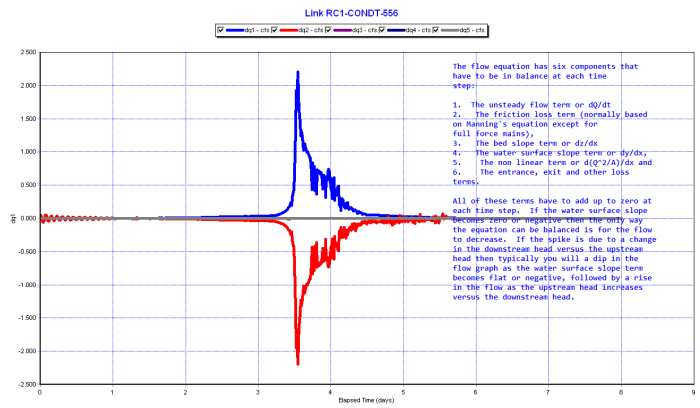#INFOSWMM

The Non Linear Term in the Saint Venant Equation of SWMM 5

Subject: Non Linear Term in the Saint Venant Equation of SWMM 5

The flow equation has six components that have to be in balance at each time step:

1. The unsteady flow term or dQ/dt

2. The friction loss term (normally based on Manning's equation except for full force mains),

3. The bed slope term or dz/dx

4. The water surface slope term or dy/dx,

5. The non linear term or d(Q^2/A)/dx and

6. The entrance, exit and other loss terms.

All of these terms have to add up to zero at each time step. If the water surface slope becomes zero or negative then the only way the equation can be balanced is for the flow to decrease. If the spike is due to a change in the downstream head versus the upstream head then typically you will a dip in the flow graph as the water surface slope term becomes flat or negative, followed by a rise in the flow as the upstream head increases versus the downstream head.

You get more than the normal flow based on the head difference because in addition to the head difference you also get a push from the nonlinear terms or dq3 and dq4 in this graph.via Blogger http://www.swmm5.net/2013/08/the-non-linear-term-in-saint-venant.html